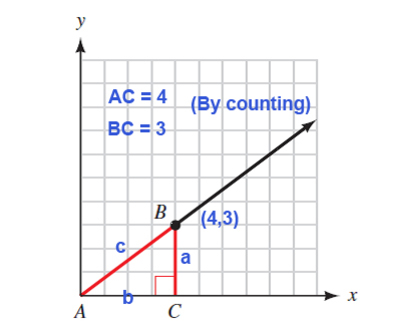## Trigonometry 7th Edition

Coordinates of point B- (4,3) Asked T-functions- $\sin A = \frac{3}{5}$ $\cos A = \frac{4}{5}$ $\tan A = \frac{3}{4}$
Steps to solution- Given that angle A is in standard position- Length AC = 4 Therefore x-coordinate of point B is '4' as it is the distance traveled along positive direction of x-axis. Length BC = 3 Therefore y-coordinate of point B is '3' as it is the distance traveled along positive direction of y-axis. Hence (4,3) are the coordinates of point B. Solution for T-functions- Considering triangle ABC right angled at C BC = 3 = a AC = 4 = b (concluded from above solution) Using this data and Pythagoras Theorem to solve for 'c'- $c^{2} =a^{2} + b^{2}$ ( Pythagoras Theorem) $c^{2} = 3^{2} + 4^{2}$ $c^{2} = 9 + 16$ $c^{2} = 25$ c = 5 Now we can write the asked T-functions of A using a = 3, b=4 and c = 5 $\sin A = \frac{a}{c} = \frac{3}{5}$ $\\cos A = \frac{b}{c} = \frac{4}{5}$ $\\tan A = \frac{a}{b} = \frac{3}{4}$